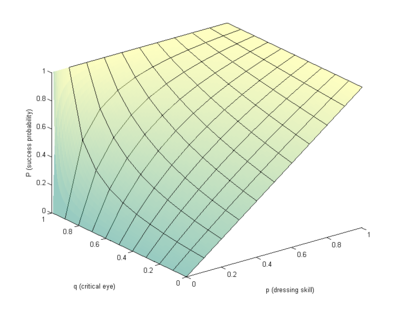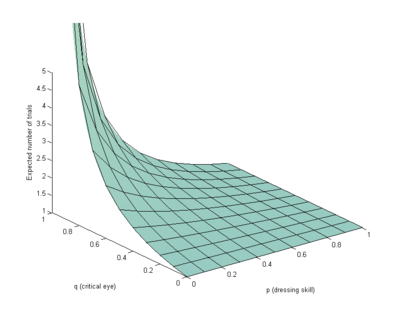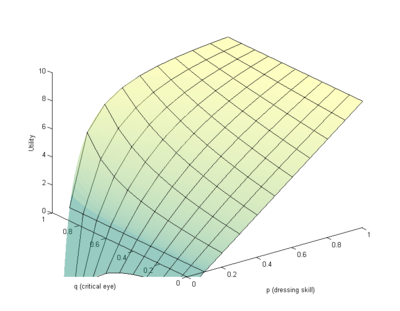## May 16, 2013

### Me on the death panel

I participated in a panel discussion at the Oxford Literary Festival earlier this year on the topic of death and the future. Now the video is up, and you can see me talking cryonics, posthumans, gods and gadgets.

Posted by Anders3 at 11:22 PM | Comments (0)

## May 15, 2013

### A mathematical model of dressingHow much time should you spend selecting clothes in the morning?

XKCD pointed out that for everyday tasks it might be rational to spend a few hours to shave off a minute each day: over the next 5 years the time will be saved. Of course, to some people dressing is a delight that should not be unduly shortened. But many of my friends find it a chore: they might recognize the importance of being decently dressed, yet find it hard to do. Hence they agonize over it, and do not find it a very worthwhile activity.

In the following I will show that it pays off to learn to dress better, but maybe not so much being an overly critical dresser.

Suppose you have probability p of selecting something that looks good, and probability q of noticing when you have a bad combination. So after the first try you have a nice combination with probability p, leave with something ugly with probability (1-p)(1-q), and do a new try with probability (1-p)q. Then the total probability of ending up with something nice will be P = p + (1-p)q( p + (1-p)q ( p + ... )))). The series S=1+x(1+x(1+...)))) must fulfil S=1+x(S), or S=1/(1-x) (it is a geometric series after all), so P = p/(1-q(1-p)).

For example, if p=0.5 and q=0.25, P=0.5/(1-.125)=0.57. If you have a sharper eye, q=0.5, and now P=0.66. Being good at selecting clothes (p) obviously helps, but noticing clashes (q) can compensate for bad selection ability - it just takes time.How long does it take? The number of steps is distributed as a geometric random variable with parameter (1-p)q. So you should expect to do 1/(1-(1-p)q) trials before you finish. In the above p=q=0.5 case you would hence on average try 1.33 times. Just being critical will not slow you down if you are good at selecting, but if you are bad at selecting yet critical it will take a while.What is the most efficient level of critical scrutiny? We could model the utility as the probability of dressing nicely divided by the number of steps it takes:
U= [p/(1-(1-p)q)] / [1/(1-(1-p)q)] = p. So by this measure critical scrutiny doesn't matter, only your basic skill in selecting clothes.

Another utility model says that nice appearance has value N and sloppy appearance has zero value, and lost time has value -t per step. In that case the total utility will be
U=N(p/(1-q(1-p))) - t/(1-q(1-p)) = (Np - t)/(1-q(1-p))
So the utility goes up for higher q if N>t/p. If the value of looking neat across the day is better than losing a few minutes in the morning you should hence be a critical dresser. If if the value isn't high, just throw on anything that fits and is clean enough.In fact, most of us likely value avoiding embarrassment more than losing a bit of time. So if we add a -N value penalty term for looking bad, we get U= (N(2p-1) - t)/(1-q(1-p)). This function varies much more with p than q, suggesting that if embarrassment is a major factor in your thinking besides efficiency you should aim at becoming better at selecting clothes in the first place - being highly critical might actually not help a lot.

Conclusions: By thinking through these considerations now, you can optimize your dressing for the foreseeable future. You can save a lot of time by increasing p, especially if it is really low (it is both likely easy to increase, and it reduces the time spent iterating - I recommend "Dress for Success" by John T. Molloy as a classic intro, relevant even if you are not a businessman). Being overly critical is of much less use than it seems: yes, sometimes you will spot a stupid mistake, but becoming better at p helps more than boosting q.

In situations where variation doesn't matter, it might be best to use memoization: just spend enough time to find a really perfect combination (or a set of combinations) and store them for later. That way dressing can be solved in O(1) time for everyday use.

(This post was originally a reply on the Extropians mailing list, now extended and corrected)

Posted by Anders3 at 10:23 AM | Comments (0)